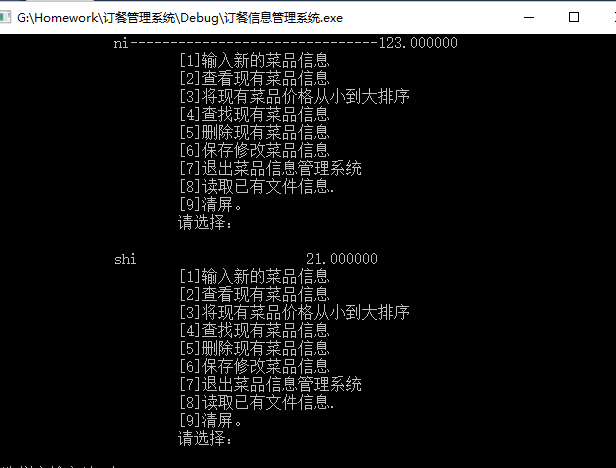c语言读写文件时出现的问题---------一下是源码

#include
#include
#define N 30
#include
struct recipe{
char food;
float price;
struct recipe *next;

};

struct recipe* creat()
{ int x;
int i=0;
printf("请输入新添加的菜品数量:");
scanf("%d",&x);
for(i=0;i p=(struct recipe *)malloc(sizeof(struct recipe));
if(p==NULL)
{ printf("分配内存失败.");
exit(1);
}
p->next=NULL;
printf("请输入菜品的名称:");
scanf("%s",p->food);
printf("请输入菜品价格:");
scanf("%f",&p->price);

``````if(head==NULL)
{
tail=p;
}
else
{  tail->next=p;
tail=p;
}
}
``````

}
void displayRecipe(struct recipe *p)
{

printf("\t\t菜名--------------------------------价格\n");
while(p!=NULL)
{

printf("\t\t%s-------------------------------%f\n",p->food,p->price);
p=p->next;
}

}

``````printf("\t\t\t输入新的菜品信息\n");

printf("\t\t\t查看现有菜品信息\t\n");

printf("\t\t\t将现有菜品价格从小到大排序\t\n");

printf("\t\t\t查找现有菜品信息\t\n");

printf("\t\t\t删除现有菜品信息\t\n");

printf("\t\t\t保存修改菜品信息\t\n");

printf("\t\t\t退出菜品信息管理系统\t\n");

printf("\t\t\t读取已有文件信息.\t\n");

printf("\t\t\t清屏。\t\n");

printf("\t\t\t请选择：    \n");

return  0;
``````

}

struct recipe *Newsth(struct recipe *p)
{
struct recipe *m;
if((m=(struct recipe *)malloc(sizeof(struct recipe)))==NULL)
{
printf("分配内存失败");
exit(1);
}

``````printf("请输入新输入的菜品名称:");
scanf("%s",m->food);
printf("请输入新输入的菜品价格:");
scanf("%f",&m->price);
m->next=p;
return m;
``````

}

struct recipe *sort(struct recipe *b)
{
struct recipe *p,*pre_min,*min,*first,*tail;
first=NULL;
while(b->next!=NULL)
{
for(p=b,min=b;p->next!=NULL;p=p->next)
{
if((p->next->price)<(min->price))
{
pre_min=p;//保留当前所找到最小节点的前驱节点
min=p->next;//保留当前最小节点
}

``````    }
if(b==min)
{
b=b->next;
}
else
{
pre_min->next=min->next;//将当前最小节点移出链表
}
if(first==NULL)
{
first=min;
tail=first;
}
else
{
tail->next=min;
tail=min;

}

}

if(b->next==NULL)
{
tail->next=b;
tail=b;

}
return first;
``````

}

void search(struct recipe *d)
{ char name;
printf("请输入需要查找的菜品名称:");
scanf("%s",name);
while(strcmp(d->food,name)!=0)
{
d=d->next;
if(d==NULL){
printf("输入有误，请重新输入.\n");
break;}
}
if(d!=NULL) printf("你要查找的菜品信息为%s,价格为%f\n",d->food,d->price);

}

struct recipe *Delete(struct recipe *h)
{ struct recipe *f,*j,*start;
char target;
start=h;
start->next=h->next;
printf("请输入你想要删除的菜品的名称:");
scanf("%s",target);

``````while(strcmp(h->food,target)!=0)
{
if(h->next->next==NULL)
j=h;

h=h->next;

}

if(h->next==NULL)
{
j->next=NULL;
free(h);

}

else{
strcpy(h->food,h->next->food);
h->price=h->next->price;
f=h->next;
if(h->next->next!=NULL)
h->next=h->next->next;
else h->next=NULL;
free(f);
}
return start;
``````

}
void save(struct recipe *g)
{
FILE *fp;
if((fp=fopen("d:\recipe.txt","w"))==NULL)
{
printf("无法打开文件.\n");
exit(0);
}

``````while(g!=NULL)
{
fprintf(fp,"\t\t%s\t\t\t%f\n",g->food,g->price);
g=g->next;
}
fclose(fp);
``````

}

``````FILE *f;
if((f=fopen("d://recipe.txt","r"))==NULL)
{
printf("无法读取文件.\n");
exit(0);
}
while(j!=NULL)
{
fscanf(f,"\t\t%s\t\t\t%f\n",j->food,&j->price);

printf("\t\t%s\t\t\t%f\n",j->food,j->price);
j=j->next;
}

fclose(f);
``````

}

void cls()
{
system("cls");
}

void main(){
int n=0;

``````struct recipe *head=NULL;

printf("--------------------欢迎进入订餐管理系统--------------------------\n");
printf("-------首次进入请确认你已写好菜品信息--------\n");
system("cls");

while(n!=7){
scanf("%d",&n);
switch(n){
break;
break;
break;
break;
break;
break;
break;
break;
case  9:cls();
break;
printf("输入有误，请重新输入.\n");

}
}
system("pause");
``````

}

3个回答

`````` void read(struct recipe *j)
{
struct recipe *p,*p1,*p2;//添加三个结构体指针
FILE *f;
if((f=fopen("d://recipe.txt","r"))==NULL)
{
printf("无法读取文件.\n");
exit(0);
}
//读取多行

p=(struct recipe *)malloc(sizeof(struct recipe));//新开辟空间
j->next=p;
p1=p;
while (2==fscanf(f,"\t\t%s\t\t\t%f\n",p1->food,&p1->price))//读取文件中的数据，每次读取一行
{
printf("\t\t%s\t\t\t%f\n",p1->food,p1->price);//打印
//新开辟一个空间，然后再来读取，并连接到原有链表上
p=(struct recipe *)malloc(sizeof(struct recipe));
p2=p1;
p1->next=p;
p1=p;
}
free(p);//释放无用指针
p=NULL;
p2->next=NULL;
fclose(f);
}
``````

{
struct recipe *p,*p1,*p2;//添加三个结构体指针
FILE *f;
if((f=fopen("d://recipe.txt","r"))==NULL)
{
printf("无法读取文件.\n");
exit(0);
}
//读取多行

``````p=(struct recipe *)malloc(sizeof(struct recipe));//新开辟空间
while(j->next!=NULL)
j=j->next;//这样就可以实现将上次保存的数据直接读取到现在建立的链表中去，实现一个多次运用
j->next=p;
p1=p;
while (2==fscanf(f,"\t\t%s\t\t\t%f\n",p1->food,&p1->price))//读取文件中的数据，每次读取一行
{
printf("\t\t%s\t\t\t%f\n",p1->food,p1->price);//打印
//新开辟一个空间，然后再来读取，并连接到原有链表上
p=(struct recipe *)malloc(sizeof(struct recipe));

p2=p1;
p1->next=p;
p1=p;
}
free(p);//释放无用指针
p=NULL;
p2->next=NULL;
fclose(f);
``````

}3 年多之前 回复3 年多之前 回复Linux下 C语言 读写 块设备文件 问题

c语言文件读写遇到的问题

``` #include <stdio.h> #include <stdlib.h> int main() { FILE *fp;FILE *fp2; fp=fopen(".//file.txt","w"); fprintf(fp,"???????????????"); fclose(fp); fp2=fopen(".//file.txt","r"); printf("0"); while(!feof(fp2)) { printf("1"); } fclose(fp2); return 0; } ``` 该程序一运行就一直输出1，我在另一个程序也遇到这个问题，需要尽快解决，谢谢各位

C语言大量文件依次读写

c语言文件读写问题，每次写入文件指针就出现错误

#include<stdio.h> #include<string.h> #include<stdlib.h> int main() { int i = 0; char b; char a[] = "iloveyou"; printf("%s\n", a); FILE *fp=NULL; char *filename = "c:/1.txt"; fp = fopen(filename, "a+"); if (fp == NULL) { printf("func fopen() err\n"); return -1; } for (i = 0; i<strlen(a); i++) { fputc(a[i], fp);//写入 //每次这里就出错 } for (i = 0; i < strlen(a); i++) { if (!feof(fp)) { b = fgetc(fp); printf("%c", b); } } fclose(fp); getchar(); return 0; } //iloveyou //屯屯屯屯 //这是结果

C语言文件读写读取不了

c语言中文件的读取问题。

#include<stdio.h> #include<string.h> #include<stdlib.h> #define N 1 int main() { struct call { char name; char telephone; char type; }; struct call s1[N]; int i; FILE *fp; char filename; fp=fopen(filename,"w"); printf("请输入打开路径:\n"); gets(filename); if(fp==NULL) { printf("此路径打不开文件\n"); exit (0); } for(i=0;i<=N;i++) { printf("请输入第%d个人的信息\n",i+1); printf("请输入联系人的姓名:\n"); gets(s1[i].name); printf("请输入联系人的电话:\n"); gets(s1[i].telephone); printf("请输入联系人的类别:\n"); gets(s1[i].type); fwrite(&s1[i],sizeof(struct call),1,fp); } fclose(fp); fp=fopen(filename,"rt"); printf("请输入打开路径:\n"); gets(filename); printf("通讯录的数据为:\n"); printf("姓名 电话号码 类别\n"); i=0; while(fread(&s1[i],sizeof(struct call),1,fp)!=NULL) { printf("%s %s %s\n",s1[i].name,s1[i].telephone,s1[i].type); i++; } fclose(fp); return 0; } 将数据写去文件时，终端可以显示，但顺着路径找，文件里面没有，刚开始学习文件，所以有点不知所措，望指导指导！

c语言文件读写操作的问题

C语言，文件问题，最后出现乱码

C语言读取文件时候的路径问题

c语言文件读写的问题，为什么读出的字节会变长

C语言 文件读写fscanf 不能读取文件第一行

``` #include<stdio.h> #include <stdlib.h> #include<conio.h> #include<String.h> int main() { FILE *fp; char ch; char zeng; fp=fopen("F:\\啦啦.txt","at+"); if((fp=fopen("F:\\啦啦.txt","at+"))==NULL) { printf("Cannot open file strike any key exit!"); getch(); exit(1); } ch=fgetc(fp); while (ch != EOF) { putchar(ch); ch=fgetc(fp); } //这个过程是进行读数据的测试过程 //接下来进行写数据 scanf("%s",zeng); fputs(zeng,fp); fclose(fp); system("pause"); return 0; } ``` 我想完成文件的读写，我用的window10系统，在F盘建立文件啦啦.txt。想对它通过C语言练习文件读写。上面代码完成了。但是有个问题，就是写数据，最多能写20个字符。我不想用这种方式，因为假如我只写3个字符，就浪费了些空间，写22个字符的话，就溢出了，有没有办法能兼容这两个问题，灵活的写任意长度的字符都没事呢

![图片说明](https://img-ask.csdn.net/upload/201512/27/1451228969_853249.png) ``` #include<stdio.h> #include<string.h> int main() {char a={0},*p,*temp; int i=0,j,k; FILE *fp=fopen("11.25","r"); while(fscanf(fp,"%s",a[i++])!=EFO); fclose(fp); for(j=0;j<i;j++) p[j]=&a[j]; for(j=0;j<i;j++) for(k=j+1;k<i;k++) if(strcmp(p[j],p[k])>0) temp=p[j],p[j]=p[k],p[k]=temp; for(k=0;k<i;k++) puts(p[k]); } ``` 请问我的代码为什么无法运行出来结果 应该咋改？

C语言文件读取写入的问题

C语言二进制文件读写异常

``` #include <stdio.h> int main() { FILE* fp = fopen("D:\\计算机\\VSdemo\\CPUdemo\\dict.dic2", "rb"); unsigned int x; fread(&x, sizeof(x), 1, fp); printf("%u", x); } ``` 想写一个读取二进制数的程序，文件中的二进制数是00001011000100000000000000000000，输出是808464432，实在不明白

c语言 大量数据在写入文件时会崩溃 急求问题所在和解决办法

String s = new String(" a ") 到底产生几个对象？

Linux面试题（2020最新版）

JVM内存结构和Java内存模型别再傻傻分不清了

JVM内存结构和Java内存模型都是面试的热点问题，名字看感觉都差不多，网上有些博客也都把这两个概念混着用，实际上他们之间差别还是挺大的。 通俗点说，JVM内存结构是与JVM的内部存储结构相关，而Java内存模型是与多线程编程相关，本文针对这两个总是被混用的概念展开讲解。 JVM内存结构 JVM构成 说到JVM内存结构，就不会只是说内存结构的5个分区，而是会延展到整个JVM相关的问题，所以先了解下

loonggg读完需要3分钟速读仅需 1 分钟大家好，我是你们的校长。我之前讲过，这年头，只要肯动脑，肯行动，程序员凭借自己的技术，赚钱的方式还是有很多种的。仅仅靠在公司出卖自己的劳动时...

85后蒋凡：28岁实现财务自由、34岁成为阿里万亿电商帝国双掌门，他的人生底层逻辑是什么？...

MySQL数据库面试题（2020最新版）

HashMap底层实现原理，红黑树，B+树，B树的结构原理 Spring的AOP和IOC是什么？它们常见的使用场景有哪些？Spring事务，事务的属性，传播行为，数据库隔离级别 Spring和SpringMVC，MyBatis以及SpringBoot的注解分别有哪些？SpringMVC的工作原理，SpringBoot框架的优点，MyBatis框架的优点 SpringCould组件有哪些，他们...